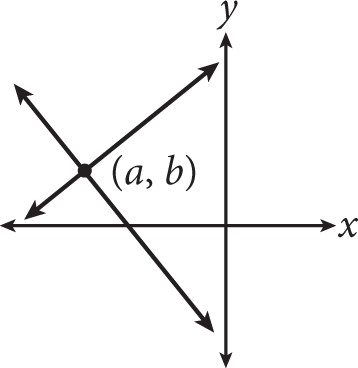# SAT Math Multiple Choice Question 377: Answer and Explanation

### Test Information

Question: 377

2.If (a, b) represents the solution to the system of equations shown in the graph and a = -3b, then which of the following could be the value of a + b?

• A. -9
• B. 0
• C. 3
• D. 6